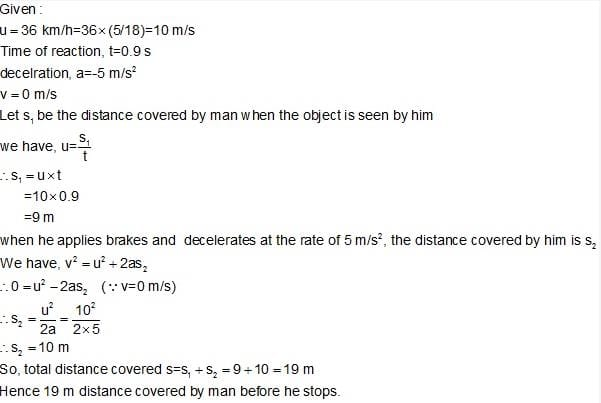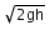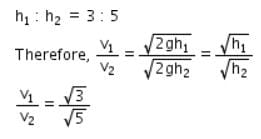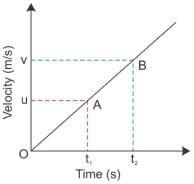MCQ: Equations of Motion

# MCQ: Equations of Motion - Class 9

Test Description

## 10 Questions MCQ Test Science Class 9 - MCQ: Equations of Motion

MCQ: Equations of Motion for Class 9 2023 is part of Science Class 9 preparation. The MCQ: Equations of Motion questions and answers have been prepared according to the Class 9 exam syllabus.The MCQ: Equations of Motion MCQs are made for Class 9 2023 Exam. Find important definitions, questions, notes, meanings, examples, exercises, MCQs and online tests for MCQ: Equations of Motion below.
Solutions of MCQ: Equations of Motion questions in English are available as part of our Science Class 9 for Class 9 & MCQ: Equations of Motion solutions in Hindi for Science Class 9 course. Download more important topics, notes, lectures and mock test series for Class 9 Exam by signing up for free. Attempt MCQ: Equations of Motion | 10 questions in 10 minutes | Mock test for Class 9 preparation | Free important questions MCQ to study Science Class 9 for Class 9 Exam | Download free PDF with solutions
 1 Crore+ students have signed up on EduRev. Have you?
MCQ: Equations of Motion - Question 1

### A man is moving with 36 kmph. The time of reaction is 0.9 seconds. On seeing an obstacle in the path, he applies brakes and decelerates at 5 m/s2, the total distance covered before he stops is:

Detailed Solution for MCQ: Equations of Motion - Question 1MCQ: Equations of Motion - Question 2

### The ratio of the heights from which two bodies are dropped is 3:5 respectively. The ratio of their final velocities is:​

Detailed Solution for MCQ: Equations of Motion - Question 2

Velocity acquired by the two bodies falling from rest through a distance his given as v =Given that :MCQ: Equations of Motion - Question 3

### A body starts to slide over a horizontal surface with an initial velocity of 0.2 m/s. Due to friction, its velocity decreases at the rate of 0.02 m/s2. How much time will it take for the body to stop?

Detailed Solution for MCQ: Equations of Motion - Question 3
Initial velocity, u = 0.2 m/s.
Final velocity, v = 0
Acceleration, a = 0.02 m/s^2.
v = u + at
0 = 0.2 - 0.02 x t
0.02 x t = 0.2
t = 0.2/0.02 = 10 sec.
Thus, the body will take 10 seconds to stop.
MCQ: Equations of Motion - Question 4

If you whirl a stone on the end of the string and the string suddenly breaks, the stone will:

Detailed Solution for MCQ: Equations of Motion - Question 4
The correct option is A because when a stone is whirling around ,tied to a string its direction of the motion is constantly changing ,even if the speed/velocity is uniform.At the time of the string breaking,the stone because of it inertia of motion follows the direction which it had at the time of string breaking,which is mostly the tangent.
MCQ: Equations of Motion - Question 5

To describe the motion of an object we first specify a

Detailed Solution for MCQ: Equations of Motion - Question 5
Yes this is because we need to observe the motion of object or rest of object from any point called reference point.
MCQ: Equations of Motion - Question 6

The third equation of motion is

Detailed Solution for MCQ: Equations of Motion - Question 6

The third equation of motion is :-
( v² - u² = 2as)
Here,
u = initial velocity
v =  final velocity
a =  acceleration
s =  distance

MCQ: Equations of Motion - Question 7

The time taken by a train to slow down from 80 kmh-1 to 20 kmh-1 with a uniform deceleration of 2 ms-2 is

Detailed Solution for MCQ: Equations of Motion - Question 7

v= 80*1000/3600 = 22.22 u=20*1000/3600=5.55 , Now a = v-u/t = 22.22-5.55/2 = 8.335

MCQ: Equations of Motion - Question 8

The equations of motion can be derived by using:

Detailed Solution for MCQ: Equations of Motion - Question 8

Consider a velocity - time graph for a uniformly accelerated body starting from rest is represented as follows.u = velocity at time t1
v =  velocity at time t2
If acceleration is represented as a, then, acceleration is defined as the rate of change in velocity.
⇒  a = v-u / t2 - t1
⇒ a = v-u /t
Or,v-u = at
⇒ v= u + at

MCQ: Equations of Motion - Question 9

A racing car has a uniform acceleration of 6 m/s2. In 10s it will cover:​

Detailed Solution for MCQ: Equations of Motion - Question 9

Given:Acceleration = 6 m/s^2Time = 10 secAs car start from rest so intial velocity will be zero.u = 0S = ut + 1/2(at^2)S = 0 + 1/2 x 6 x 100S = 300 m.

MCQ: Equations of Motion - Question 10

A body performs an accelerated motion, with uniform speed. The motion of body is

Detailed Solution for MCQ: Equations of Motion - Question 10
This is because in circular motion body is continuously changing its speed and acceleration is the change of velocity.

## Science Class 9

66 videos|352 docs|97 tests
Information about MCQ: Equations of Motion Page
In this test you can find the Exam questions for MCQ: Equations of Motion solved & explained in the simplest way possible. Besides giving Questions and answers for MCQ: Equations of Motion, EduRev gives you an ample number of Online tests for practice

## Science Class 9

66 videos|352 docs|97 tests